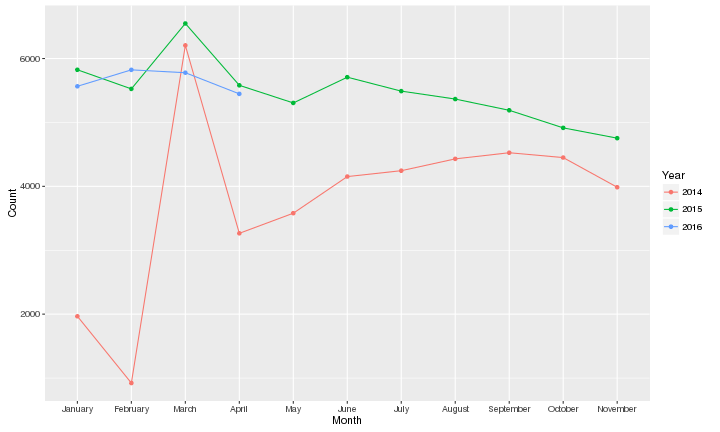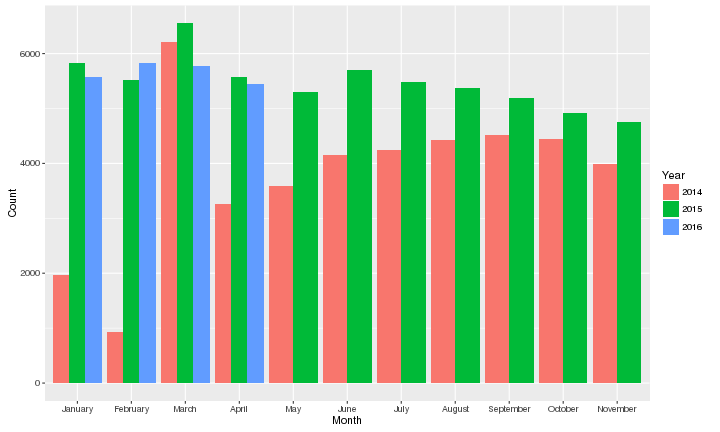# GitHub R Repository Counts by Month

Illinois State University (IT497)

## Research Question

Are there more GitHub R repositories created in February than January?

## Preparation

These are the various libraries needed when executing this code.

``````library(ggplot2)
library(RCurl)
library(rjson)
library(stringr)
``````

These are the variables that you'll need to put your GitHub API client ID and client secret into.

``````client.id <- "GITHUB_ID_HERE"
client.secret <- "GITHUB_SECRET_HERE"
``````

## Obtaining/Scrubbing Data

This function defines a generic function for using the GitHub API. It will take in a search type, query, and page number (optional). The return is the decoded JSON as returned by the rjson::fromJSON() function.

search_type - One of “repositories”, “code”, “issues”, or “users”.

q - Query as defined on: https://help.github.com/articles/search-syntax/

page - Optional page number for query results as defined here: https://developer.github.com/v3/#pagination

``````gh_search <- function(search_type, q, page=1){
# this prevents issues with rate limiting
# (GitHub has 30 requests per minute limit)
Sys.sleep(5)

# now perform query
fromJSON(getURL(paste0(
"https://api.github.com/search/",
search_type,
"?client_id=", client.id,
"&client_secret=", client.secret,
"&q=", curlEscape(q),
"&page=", page
}
``````

This function defines a search for a total number of results by language, year, and month. Returns the total number as a single number if only one month is provided or as a vector of results if multiple months are provided.

language - The language name to search for.

year - The year to search for.

month - The month or vector of months to search. If multiple months are provided, they will be looped through and returned as a vector accordingly.

``````gh_lang_date <- function(language, year, month){
ret <- NULL
# loop given months
for(begin_month in month){
end_month <- as.integer(begin_month)+1
end_date <- 1

# adjust end month if we are dealing with December
if(end_month > 12){
end_month <- 12
end_date <- 31
}

# pad values for string based search result
begin_month <- str_pad(begin_month, 2, "left", "0")
end_month <- str_pad(end_month, 2, "left", "0")
end_date <- str_pad(end_date, 2, "left", "0")

# construct search query and conduct search
result <- gh_search("repositories", paste0(
"language:", language,
' created:"',
year, "-", begin_month, "-01 .. ",
year, "-", end_month, "-", end_date,
'"'
))\$total_count

# append result to return value
ret <- c(ret, result)
}
# return results
ret
}
``````

This command searchs for the R programming language for 2014 between January and December.

``````data_2014 <- gh_lang_date("R", 2014, 1:12)
``````

This command searchs for the R programming language for 2015 between January and December.

``````data_2015 <- gh_lang_date("R", 2015, 1:12)
``````

This command searchs for the R programming language for 2016 between January and April. At time of writing, April 2016 is the last full month.

``````data_2016 <- gh_lang_date("R", 2016, 1:4)
``````

This command produces a data frame that combines the counts together, then generates a set of POSIXlt dates associated with those counts.

``````final_data <- data.frame(
Count=c(data_2014, data_2015, data_2016),
Date=as.POSIXlt(paste0(
c(
rep(2014, length(data_2014)),
rep(2015, length(data_2015)),
rep(2016, length(data_2016))
seq(1, length(data_2014)),
seq(1, length(data_2015)),
seq(1, length(data_2016))
), 2, "left", "0"), "-01"
), format="%Y-%m-%d")
)
``````

This command sorts the data by date.

``````final_data <- final_data[order(as.numeric(final_data\$Date)),]
``````

This command extracts the full month name from the POSIXlt date and converts it to a factor.

``````final_data\$Month <- strftime(final_data\$Date, format="%B")
final_data\$Month <- factor(final_data\$Month, levels=unique(final_data\$Month))
``````

This command extracts the year from the POSIXlt date and puts it in its own column, and converts it to a factor.

``````final_data\$Year <- strftime(final_data\$Date, format="%Y")
final_data\$Year <- factor(final_data\$Year, levels=unique(final_data\$Year))
``````

This command strips the POSIXlt date since we no longer need it since all we need is month and year. The only point of converting to POSIXlt in the first place was to get the full month names for the month field.

``````final_data\$Date <- NULL
``````

## Data Exploration

#### Exploration

``````class(final_data)
``````
``````##  "data.frame"
``````
``````str(final_data)
``````
``````## 'data.frame':    26 obs. of  3 variables:
##  \$ Count: num  1967 922 6206 3265 3580 ...
##  \$ Month: Factor w/ 11 levels "January","February",..: 1 2 3 4 5 6 7 8 9 10 ...
##  \$ Year : Factor w/ 3 levels "2014","2015",..: 1 1 1 1 1 1 1 1 1 1 ...
``````
``````summary(final_data)
``````
``````##      Count           Month      Year
##  Min.   : 922   January : 3   2014:11
##  1st Qu.:4291   February: 3   2015:11
##  Median :5248   March   : 3   2016: 4
##  Mean   :4790   April   : 3
##  3rd Qu.:5577   May     : 2
##  Max.   :6549   June    : 2
##                 (Other) :10
``````

## Results

#### Data

The data contains three columns, one for the year, one for the month, and one for the count of R repositories created in the associated year and month fields.

``````final_data
``````
``````##    Count     Month Year
## 1   1967   January 2014
## 2    922  February 2014
## 3   6206     March 2014
## 4   3265     April 2014
## 5   3580       May 2014
## 6   4153      June 2014
## 7   4245      July 2014
## 8   4430    August 2014
## 9   4526 September 2014
## 10  4450   October 2014
## 11  3986  November 2014
## 12  5824   January 2015
## 13  5525  February 2015
## 14  6549     March 2015
## 15  5581     April 2015
## 16  5305       May 2015
## 17  5708      June 2015
## 18  5490      July 2015
## 19  5366    August 2015
## 20  5190 September 2015
## 21  4915   October 2015
## 22  4754  November 2015
## 23  5566   January 2016
## 24  5823  February 2016
## 25  5778     March 2016
## 26  5447     April 2016
``````

#### Analysis

Assuming 2016 is the year being compared, there were 5566 R repositories created in January and 5823 R repositories created in February, so there were more R repositories created in February 2016.

#### Graphs

``````g <- ggplot(final_data, aes(x=Month, y=Count, group=Year, color=Year))
g + geom_line() + geom_point()
````````````g <- ggplot(final_data, aes(x=Month, y=Count, group=Year, fill=Year))
g + geom_bar(stat="identity", position="dodge")
``````